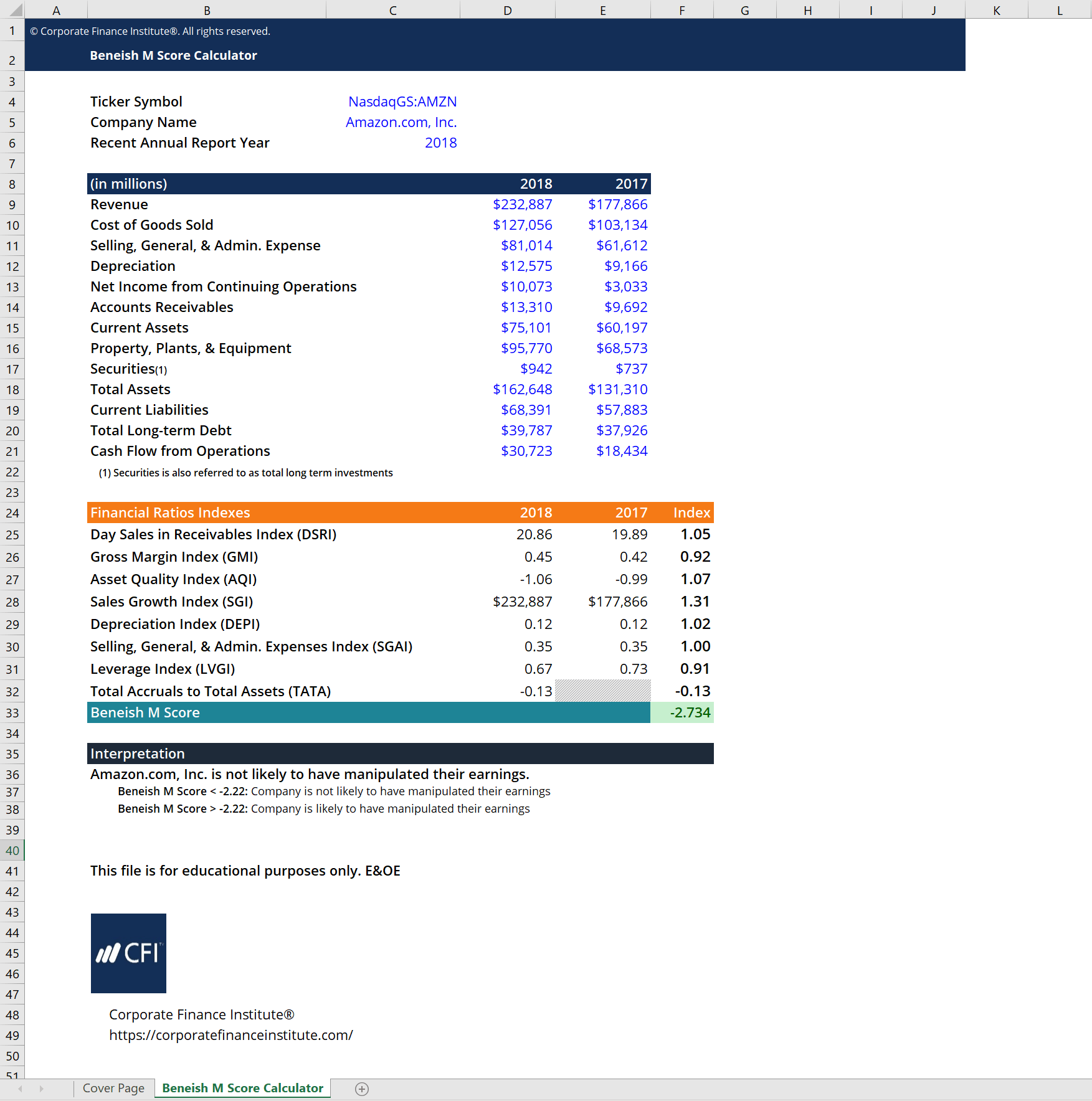# Beneish M Score Calculator

## Beneish M Score Calculator

The Beneish M Score calculator is used to determine whether or not the company has manipulated its reported earnings in its financial statements. This Beneish M Score calculator takes financial data that can be found on the company’s financial statements as inputs and calculates eight different financial ratios. It then applies the M score formula to these ratios, giving different weights to each one to calculate a final predictive score.

Below is a preview of CFI’s Beneish M Score calculator:### Ratios and Formula

The Beneish M score calculator uses eight different ratios when calculating its conclusion. These ratios are as follows:

Day Sales in Receivables Index (DSRI): (Accounts Receivablest/Salest*Number of Days) / (Accounts Receivablest-1/Salest-1*Number of Days)

• Note: the original formula uses ‘net receivables’ instead of ‘accounts receivables’ but it is not always easy to find this information so it is substituted with accounts receivables instead. Additionally, ‘net credit sales’ is often not available in a company’s financial statements, so ‘sales’ used instead.

Gross Margin Index (GMI): [Salest-1 – COGSt-1)/Salest-1] / [Salest – COGSt)/Salest]

Asset Quality Index (AQI): [1 – ((Current Assetst + PP&Et + Total Long-term Investmentst)/Total Assetst)] / [1 – ((Current Assetst-1 + PP&Et-1 + Total Long-term Investmentst-1) / Total Assetst-1)]

• Note: ‘total long-term investments’ is also referred to as ‘securities’.

Sales Growth Index (SGI): Salest / Salest-1

Depreciation Index (DEPI): (Depreciationt-1/(PP&Et-1 + Depreciationt-1)) / (Depreciationt/(PP&Et + Depreciationt))

Selling, General, & Admin. Expenses Index (SGAI): (SG&A Expenset/Salest) / (SG&A Expenset-1/Salest-1)

Leverage Index (LVGI): [(Current Liabilitiest + Total Long-term Debtt)/Total Assetst] / [(Current Liabilitiest-1 + Total Long-term Debtt-1) / Total Assetst-1]

Total Accruals to Total Assets (TATA): (Income from Continuing Operationst – Cash Flow from Operationst) / Total Assetst

• Note: ‘net income’ can be used instead of  ‘income from continuing operations’ if the company does not specify any sort of non-recurring income.

By examining these ratios, the Beneish M Score calculator takes into account several factors, such as the change in leverage and change in asset quality. The final formula for Beneish’s statistical model is shown below.### Interpreting the Beneish M Score

The Beneish M score calculator is very easy to interpret. The score outputted by the calculator determines whether or not the company you are evaluating is likely to have manipulated their earnings in their financial statements. However, keep in mind that this is only a predictive conclusion. The Beneish M score calculator only looks at the likelihood of a company manipulating their earnings. Thus, the calculator does not concretely define or determine whether the company is a manipulator or not.

There are two possible conclusions that can be made using the M score:

• Beneish M Score < -2.22: the company is not likely to have manipulated their earnings
• Beneish M Score > -2.22: the company is likely to have manipulated their earnings

### More Free Templates

For more resources, check out our business templates library to download numerous free Excel modeling, PowerPoint presentation, and Word document templates.

• Excel Modeling Templates
• PowerPoint Presentation Templates
• Transaction Document Templates
• CFI Template Marketplace

### Financial Analyst Certification

Become a certified Financial Modeling and Valuation Analyst (FMVA)® by completing CFI’s online financial modeling classes and training program!